## Matching Inequality Symbols

### Matching exercise

Match the items on the right to the items on the left.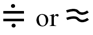??? is equal to, equals is approximately equal to is not greater than is greater than is not less than is less than is not equal to is greater than or equal to is less than or equal to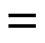??? is equal to, equals is approximately equal to is not greater than is greater than is not less than is less than is not equal to is greater than or equal to is less than or equal to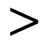??? is equal to, equals is approximately equal to is not greater than is greater than is not less than is less than is not equal to is greater than or equal to is less than or equal to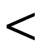??? is equal to, equals is approximately equal to is not greater than is greater than is not less than is less than is not equal to is greater than or equal to is less than or equal to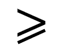??? is equal to, equals is approximately equal to is not greater than is greater than is not less than is less than is not equal to is greater than or equal to is less than or equal to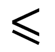??? is equal to, equals is approximately equal to is not greater than is greater than is not less than is less than is not equal to is greater than or equal to is less than or equal to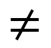??? is equal to, equals is approximately equal to is not greater than is greater than is not less than is less than is not equal to is greater than or equal to is less than or equal to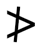??? is equal to, equals is approximately equal to is not greater than is greater than is not less than is less than is not equal to is greater than or equal to is less than or equal to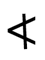??? is equal to, equals is approximately equal to is not greater than is greater than is not less than is less than is not equal to is greater than or equal to is less than or equal to